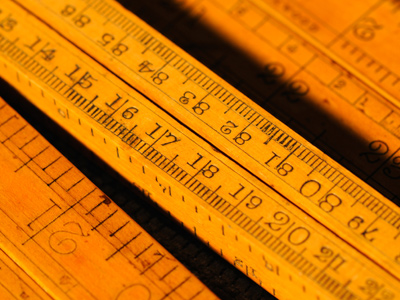You will have seen inches and centimetres on your rulers at school.

# Measures 2 (Very Easy)

This is the second of our Eleven Plus maths quizzes on measures and, like the first, it is very easy. In the previous quiz we looked in particular at metric measures, like metres, grams and tonnes. In this quiz we’ll also be investigating some old-fashioned imperial measures, like miles, inches, feet and yards.

Here are some comparisons to help you visualise:

• 1 inch = 2.54 cm
• 1 foot = 30.48 cm
• 1 yard = 91.44 cm
• 1 mile = 1,609.344 metres

Don’t worry if imperial measures seem a bit troubling. Play this quiz until you can score ten-out-of-ten, then you’ll be top of the class by a country mile!

1.
How many inches are there in a foot?
12
4
16
8
There are 12 inches in 1 foot. You may have heard of someone being 5 feet 11 inches tall, but never 5 feet 12 inches tall: that would be 6 feet
2.
How many feet are there in a yard?
10
6
5
3
There are three feet in a yard. If someone is 6 feet tall, they are also two yards tall
3.
How many yards are there in a mile?
1,000
1,760
2,000
2,100
A mile was originally 1,000 paces. Of course, people have different leg lengths, so this was standardised to 1,760 yards under the reign of Henry VIII
4.
How many square metres are there in one hectare?
100
100,000
1,000
10,000
When the metric system was introduced, the 'are' was 100 square metres. A hectare was 100 'ares', (hecto means 100, just as kilo means 1,000), 100 x 100 = 10,000 m²
5.
Metric measures are based on tens, hundreds and thousands. What are imperial measures based on?
Twelves, twenty-fours and thirty-sixes
Body parts
Henry VIII's whims
A mixture of things
Some imperial meaures, like the foot for example, are based on body parts. Others are based on seemingly random things, like the size of barley grains, the distance travelled in an hour or the amount of land an ox can plough in a day!
6.
How many inches are there in one yard?
36
12
24
48
There are 3 feet in a yard and 12 inches in a foot: 12 x 3 = 36
7.
How many feet are there in a mile?
5,280
1,760
10,560
10,000
There are 1,760 yards in a mile and 3 feet in a yard: 1,760 x 3 = 5,280
8.
If 1 inch = 2.54 cm, how many cm are in two feet?
70
60.96
50
60
There are 12 inches in 1 foot, so there must be 24 inches in 2 feet. 24 x 2.54 = 60.96.
You may have heard that there are 30 cm in 1 foot but this is an approximation. There are actually 30.48 cm in 1 foot
9.
If 1 mile = 1.6 km, how many km are in 10 miles?
18
8
16
6
To convert miles to km (approximately) multiply the number of miles by 1.6: 10 x 1.6 = 16
10.
If 1 cm = 0.39 inches, how many inches are in one metre?
400
40
390
39
Again, this is an approximation. There are actually 39.3701 inches in 1 metre - converting imperial to metric (and vice versa) is not so simple!
Author:  Frank Evans

We use cookies to make your experience of our website better.

To comply with the new e-Privacy directive, we need to ask for your consent -